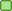###Author Topic: 2 Screens | 12 Players?  (Read 1673 times)

0 Members and 1 Guest are viewing this topic.

####rainbowdash28##### 2 Screens | 12 Players?
« on: November 11, 2018, 01:27:59 am »
Hello~

First of all: Thanks for your longtime support of this awesome game, that's incredible!
Why? Because every single year, again and again, this game is a very great part of all vienna meetups. (Besides some other games, but most of the time people wish to play MLK)

So therefor I wanted to ask something I'm going to consider a some years already: Is it possible to play the game with 12 players?
I just ask, because since some of the last updates I can choose up to 12 players, but haven't got it working yet.

Tried everything with config.ini: 2 screens, split mode (of course fullscreen off all the time), different resolutions, but still the same problem. 7-12 players won't show up but it seems like some part of it has been integrated in the game already, because it just seems to be a bit "off", like I can select player 7-12 but the cursor sticks on a side of the window, looks like it doesn't have enough space because both screens just shows the same content.

Even in the settings it seems to be prepared for 12 players.

I know, that's a really special case most people won't ask for, maybe because they don't even have the hardware to input 12 microphones at the same time without latency.

Would be great to upgrade from 6 players to more, because every single microphone is taken at the meetups and some people sing along without a microphone.I'm going to attach some screenshots which going to explain what I've tried to bring up in this text *laugh* maybe my english skills are still not the best.

Screenshots:
Select players: The cyan colored square on the left side of Rainbow Dash is the 12th player, the color of the square changes while I'm scrolling between players 7-12
Settings: There are 12 channels - that was the first hint for me that something has changed
ErrorOnQuit: That's a error which shown up when I'm going to quit the game with 7-12 players

And my config.ini:
Code: [Select]
`[Game]Players=12Difficulty=EasyLanguage=EnglishTabs=OffSongMenu=RouletteSorting=EditionShowScores=OnDebug=Off[Graphics]Screens=2Split=OffFullScreen=OffVisualization=OffResolution=1920x1080Depth=32 bitTextureSize=256SingWindow=BigOscilloscope=OnMovieSize=Full [BG+Vid]VideoPreview=OnVideoEnabled=OnMaxFramerate=60ResolutionFullscreen=1920x1080[Sound]ClickAssist=OffBeatClick=OffAudioOutputBufferSize=AutoBackgroundMusic=OnPreviewVolume=70%PreviewFading=3 SecsSavePlayback=OffVoicePassthrough=OffMusicAutoGain=Off[Lyrics]LyricsFont=OLine2LyricsEffect=SlideNoteLines=OnEncoding=Auto[Themes]Theme=MyLittleKaraokeSkin=MyLittleKaraokeColor=Blue[Record]MicBoost=+12dBThreshold=15%DeviceName=Mikrofon (2- Realtek High Definition Audio)Input=0Latency=-1Channel1=1Channel2=2DeviceName=Mix 10 (ASIOVADPRO Driver)Input=0Latency=-1Channel1=10Channel2=0DeviceName=Mix 06 (ASIOVADPRO Driver)Input=0Latency=-1Channel1=6Channel2=0DeviceName=Mix 04 (ASIOVADPRO Driver)Input=0Latency=-1Channel1=4Channel2=0DeviceName=Mix 08 (ASIOVADPRO Driver)Input=0Latency=-1Channel1=8Channel2=0DeviceName=Mix 01 (ASIOVADPRO Driver)Input=0Latency=-1Channel1=1Channel2=0DeviceName=Mix 12 (ASIOVADPRO Driver)Input=0Latency=-1Channel1=12Channel2=0DeviceName=Mix 11 (ASIOVADPRO Driver)Input=0Latency=-1Channel1=11Channel2=0DeviceName=Mix 14 (ASIOVADPRO Driver)Input=0Latency=-1Channel1=0Channel2=0DeviceName=Mix 03 (ASIOVADPRO Driver)Input=0Latency=-1Channel1=3Channel2=0DeviceName=Mix 13 (ASIOVADPRO Driver)Input=0Latency=-1Channel1=0Channel2=0DeviceName=Mix 07 (ASIOVADPRO Driver)Input=0Latency=-1Channel1=7Channel2=0DeviceName=Mikrofon (3- KAVE XTD Headset)Input=0Latency=-1Channel1=0Channel2=0DeviceName=Mix 15 (ASIOVADPRO Driver)Input=0Latency=-1Channel1=0Channel2=0DeviceName=Mikrofon (Realtek High Definition Audio)Input=0Latency=-1Channel1=0Channel2=0DeviceName=Mix 02 (ASIOVADPRO Driver)Input=0Latency=-1Channel1=2Channel2=0DeviceName=Mix 16 (ASIOVADPRO Driver)Input=0Latency=-1Channel1=0Channel2=0DeviceName=Mix 09 (ASIOVADPRO Driver)Input=0Latency=-1Channel1=9Channel2=0DeviceName=Mix 05 (ASIOVADPRO Driver)Input=0Latency=-1Channel1=5Channel2=0DeviceName=Line (BEHRINGER UMC1820)Input=0Latency=-1Channel1=0Channel2=0DeviceName=Mikrofon (4- KAVE XTD Headset)Input=0Latency=-1Channel1=0Channel2=0[Advanced]LoadAnimation=OnEffectSing=OnScreenFade=OnAskbeforeDel=OnOnSongClick=SingLineBonus=OnPartyPopup=OnSingScores=OnTopScores=AllSyncTo=MusicSingTimebarMode=RemainingJukeboxTimebarMode=Current[Controller]Joypad=OffMouse=Game[Directories]SongDir0=C:\Program Files (x86)\MyLittleKaraokeSongDir1=C:\Program Files\MyLittleKaraokeWebScoresDir=[Jukebox]LyricsFont=OLine2LyricsEffect=SlideLyricsAlpha=0.90SingLineColor=0096FFActualLineColor=A0A0A0NextLineColor=606060SingLineOColor=000000ActualLineOColor=000000NextLineOColor=000000[Name]P1=Rainbow DashP2=Twilight SparkleP3=ApplejackP4=Pinkie PieP5=FluttershyP6=RarityP7=Derpy HoovesP8=CadenceP9=Shining ArmorP10=Princess LunaP11=Princess CelestiaP12=CrackleP13=P14=P15=P16=[NameTeam]T1=Team1T2=Team2T3=Team3[NameTemplate]Name1=Template1Name2=Template2Name3=Template3Name4=Template4Name5=Template5Name6=Template6Name7=Template7Name8=Template8Name9=Template9Name10=Template10Name11=Template11Name12=Template12[PlayerColor]P1=1P2=14P3=5P4=6P5=4P6=13P7=7P8=8P9=9P10=10P11=11P12=12[PlayerAvatar]P1=8C206C471289A2C9CE6D4B192E0A3DCDP2=AC67DE538E1CE61A018C4C4DD1BC23BCP3=4BDFCAB46302F615A0D5CA64C9594FF4P4=6CBC7AE13169A6F299D18B2AB821916CP5=7758A336AE938EFBFA6969FD3FDCE595P6=560A4460BBC9658DB2E7B4CDAE13DDDBP7=P8=P9=P10=P11=P12=[PlayerLevel]P1=0P2=0P3=1P4=1P5=1P6=0P7=0P8=0P9=0P10=0P11=0P12=0[Webcam]ID=1Resolution=800x600FPS=20Flip=OnBrightness=0Saturation=0Hue=0Effect=0[TeamColor]T1=1T2=2T3=3`
Best regards & Greetings from Lower Austria; Christian / Zerix / rainbowdash28

####Renard##### Re: 2 Screens | 12 Players?
« Reply #1 on: May 04, 2019, 10:26:43 pm »
Super late answer, sorry! But that feature hasn't been fully implemented yet, so, it's not currently possible to set up the game that way.